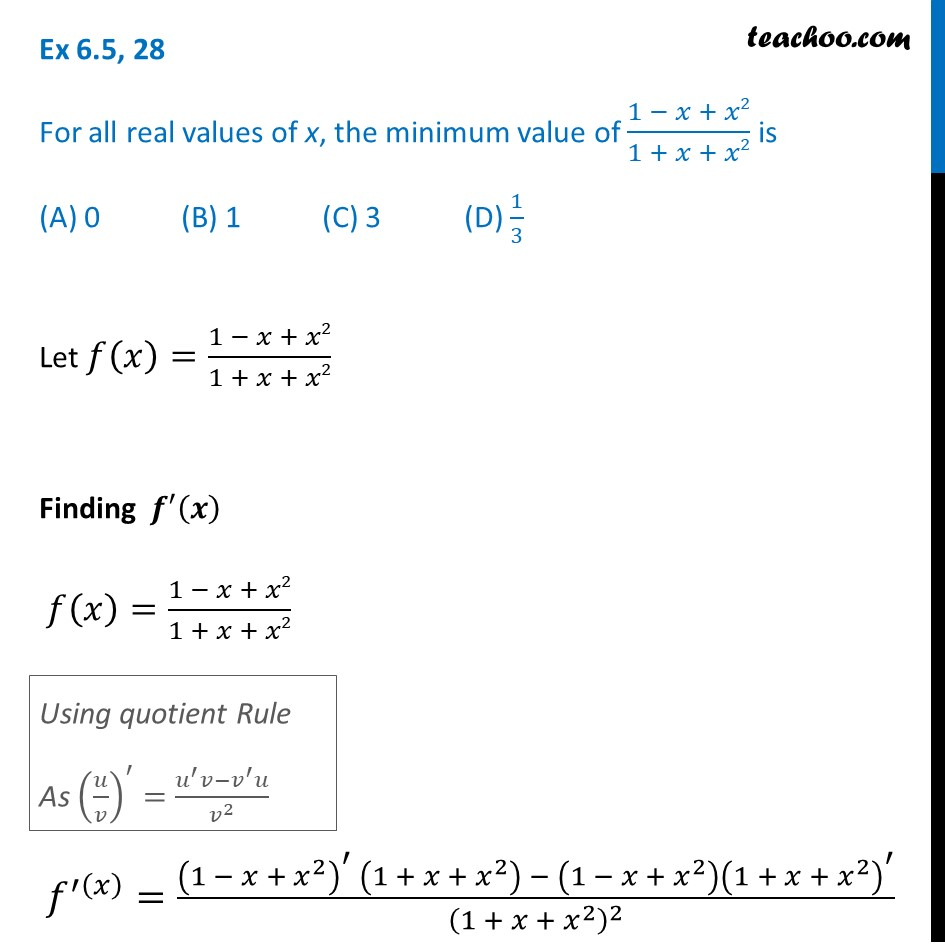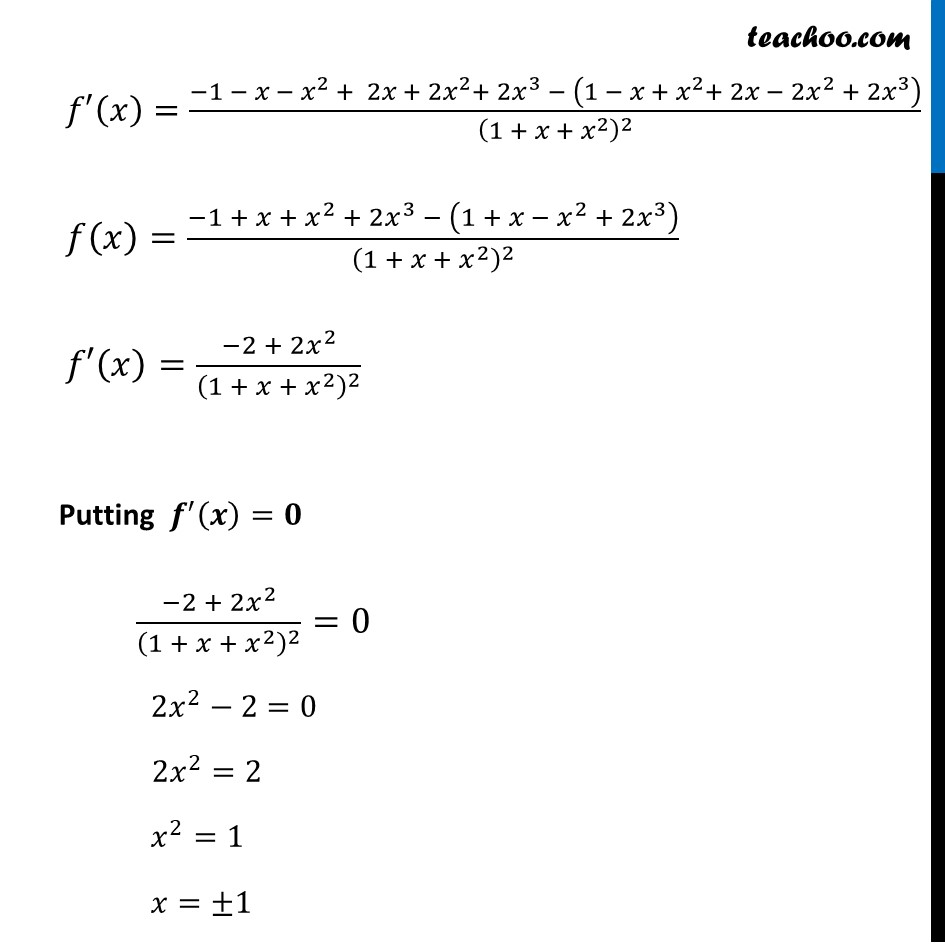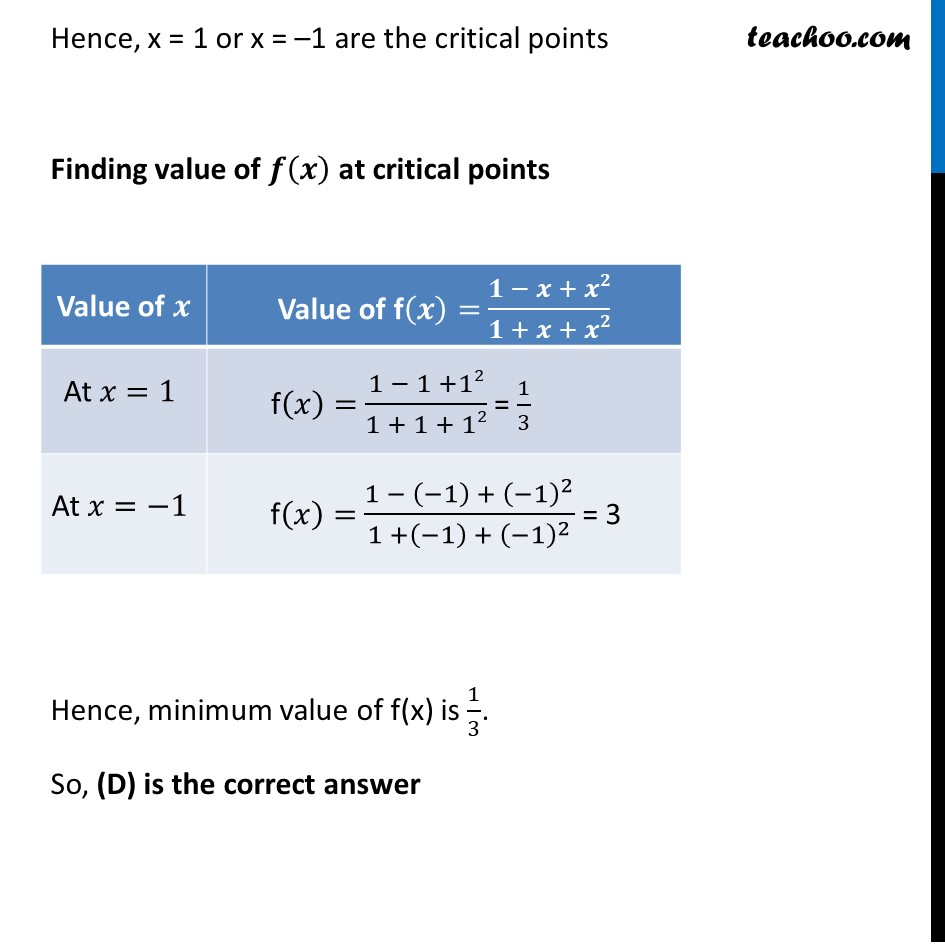1. Chapter 6 Class 12 Application of Derivatives
2. Serial order wise
3. Ex 6.5

Transcript

Ex 6.5, 28 For all real values of x, the minimum value of (1 − 𝑥 + 𝑥2)/(1 + 𝑥 + 𝑥2) is (A) 0 (B) 1 (C) 3 (D) 1/3Let 𝑓(𝑥)=(1 − 𝑥 + 𝑥2)/(1 + 𝑥 + 𝑥2) Finding 𝒇′(𝒙) 𝑓(𝑥)=(1 − 𝑥 + 𝑥2)/(1 + 𝑥 + 𝑥2) 𝑓^′(𝑥) =((1 − 𝑥 + 𝑥^2 )^′ (1 + 𝑥 + 𝑥^2 ) − (1 − 𝑥 + 𝑥^2 ) (1 + 𝑥 + 𝑥^2 )^′)/(1 + 𝑥 + 𝑥^2 )^2 Using quotient Rule As (𝑢/𝑣)^′=(𝑢^′ 𝑣−𝑣^′ 𝑢)/𝑣^2 𝑓′(𝑥)=(−1 − 𝑥 − 𝑥^2 + 2𝑥 + 2𝑥^2+ 2𝑥^3 − (1 − 𝑥 + 𝑥^2+ 2𝑥 − 2𝑥^2 + 2𝑥^3 ))/(1 + 𝑥 + 𝑥^2 )^2 𝑓(𝑥)=(−1 + 𝑥 + 𝑥^2 + 2𝑥^3 − (1 + 𝑥 − 𝑥^2 + 2𝑥^3 ))/(1 + 𝑥 + 𝑥^2 )^2 𝑓′(𝑥)=(−2 + 2𝑥^2)/(1 + 𝑥 + 𝑥^2 )^2 Putting 𝒇^′ (𝒙)=𝟎 (−2 + 2𝑥^2)/(1 + 𝑥 + 𝑥^2 )^2 =0 2𝑥^2−2=0 2𝑥^2=2 𝑥^2=1 𝑥=±1 Hence, x = 1 or x = –1 are the critical points Finding value of 𝒇(𝒙) at critical points Hence, minimum value of f(x) is 1/3. So, (D) is the correct answer

Ex 6.5